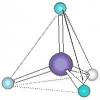BrainDen.com - Brain Teasers
• 0## QuestionIn a molecule of CF4 (carbon tetrafluoride) you have one carbon atom and four fluoride atoms. Let's assume that all four fluoride atoms are equally attracted to the carbon, and equally repelled by each other (of course it doesn't really work like that, but just work with me). What would be the angle formed by any two fluoride atoms, with the carbon atom as the vertex?

If you feel like a picture would make it easier ...## Recommended Posts

• 0
In a molecule of CF4 (carbon tetrafluoride) you have one carbon atom and four fluoride atoms. Let's assume that all four fluoride atoms are equally attracted to the carbon, and equally repelled by each other (of course it doesn't really work like that, but just work with me). What would be the angle formed by any two fluoride atoms, with the carbon atom as the vertex?
cos-1(-1/3) = 109.4712206 degrees.

Also, 2tan-1[sqrt(2)]

##### Share on other sites
• 0I think 60 degree

##### Share on other sites
• 0

Cos(ALPHA) = 1/3

ALPHA around 109 degrees

##### Share on other sites
• 0109.47 degrees

##### Share on other sites
• 0Good job, but that was too easy. This was a question that occurred to me back in high school chemistry, and I solved it using a ridiculously convoluted approach. Later I showed it to a friend and he pointed out a much simpler method, although even his solution wasn't as simple as Cos(x) = -1/3.

I'll see if I can the make the next one more challenging.

Edited by Duh Puck

##### Share on other sites
• 0
Good job, but that was too easy. This was a question that occurred to me back in high school chemistry, and I solved it using a ridiculously convoluted approach. Later I showed it to a friend and he pointed out a much simpler method, although even his solution wasn't as simple as Cos(x) = -1/3.

I'll see if I can the make the next one more challenging.

Well in fairness cos[x] = -1/3 is not a solution. It's the answer. A solution shows how the answer is obtained. I didn't do that. But for the record the formula can be derived by considering the dot product of the vectors from the carbon atom to two of the Fluorine atoms. The tangent formula can be seen by putting the carbon atom at the center of a cube. The fluorine atoms then occupy four cube corners that can be connected with face diagonals. You can easily draw a solvable triangle formed by the C atom, the midpoint of a face diagonal and an F atom at one end of that diagonal. Halve the cube diagonal and you've got the tangent of half the angle in question.

I imagine there is a solution based on spherical trigonometry as well. Anyone know of it?

##### Share on other sites
• 0Well in fairness cos[x] = -1/3 is not a solution. It's the answer. A solution shows how the answer is obtained. I didn't do that. But for the record the formula can be derived by considering the dot product of the vectors from the carbon atom to two of the Fluorine atoms. The tangent formula can be seen by putting the carbon atom at the center of a cube. The fluorine atoms then occupy four cube corners that can be connected with face diagonals. You can easily draw a solvable triangle formed by the C atom, the midpoint of a face diagonal and an F atom at one end of that diagonal. Halve the cube diagonal and you've got the tangent of half the angle in question.

Thanks! I don't feel quite as slow when you put it that way.## Join the conversation

You can post now and register later. If you have an account, sign in now to post with your account.×   Pasted as rich text.   Paste as plain text instead

Only 75 emoji are allowed.

×   Your previous content has been restored.   Clear editor

×   You cannot paste images directly. Upload or insert images from URL.

×